Study Guide

# Electromagnetism - Magnetic Field Relatives

## Magnetic Field Relatives

What else is there to talk about after Biot-Savart and Ampere’s Laws for a current-induced magnetic field? The rest of the family of these fields, of course, which just happens to be pretty large.

### Current Density

We know current is the flow of charge over time, written as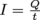and measured in amperes (A), but what exactly is current density?

Let’s take a look at the picture below: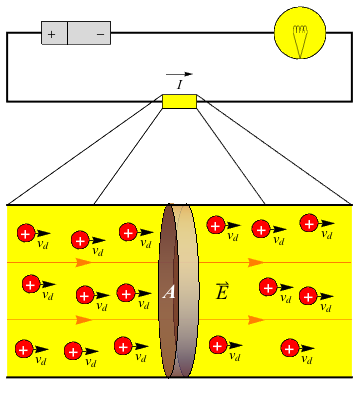Above we see the cross section of a conducting wire with current I produced by positive charges moving along an electric field E with drift velocity vd. The drift velocity is simply the average speed of the positive charges, taking into account random collisions and other factors. It may look like protons are moving, but it’s the electrons that move to form a current. The drift velocity is defined with the same magnitude for speed, but in the opposite direction as the electrons.

The conductor has n current carrying particles per unit volume V with charge q, each moving with drift velocity vd. The volume of the conductor is its cross sectional area A multiplied by its length d. There are, therefore, nAd charges in a volume V. But let’s not forget the particles each have charge q, so the total charge is ΔQ = nAdq. Taking the length d and drift velocity into account, this gives us a time of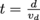.

Finally we are ready to re-write our current equation.

Now, we have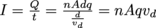.

The current density J is defined as the current per unit area at a given point in the conductor: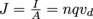.

Just like water in a pipe, the current density is the same throughout any point in the conductor with the same cross sectional area. Charge is conserved.

We could use calculus to express the current density in a more general way, but that’s not our game. We get to jump straight to the relationship between current density and (what else?) electric fields in terms of the electrical conductivity, σ: J = σE where both E and J are in the same direction because they’re vector quantities.

Yes, this unit uses σ and n, and even μ and m, in entirely different ways, so we need to pay attention to the context.

Finding another relationship with electric field is nice and all, but aren’t we supposed to be talking about magnetic fields? Stay tuned.

### Magnetic Dipole Moments

Electric dipoles have magnetic cousins. It’s true. We should have guessed it, everything else we’ve learned about in the magnetic world has a parallel in the electric world.

We’ve encountered the Lorentz force before in our studies of magnetism5 as the force that a point charge q moving at velocity v experiences while crossing a magnetic field B, in math F = qv × B. The Lorentz force involves the right hand rule because the vectors F, v, and B are all perpendicular to each other.

What if instead of a single charge crossing a magnetic field, we have a bunch of charges in a wire, a current?

We write F = nAlqv × B for the force on a wire of length l and cross-sectional area A with n charge carriers of drift velocity vd and charge q.

We’ve seen the nAlqv product before when speaking of current density. That’s the same as in I = nAqvd. Therefore, we can rewrite the magnetic force F on a current as F = Il × B.

The right-hand rule here still applies to find the direction of the magnetic force. Good old reliable right-hand rule with pointer finger along the length of the wire, the fingers curling around to the direction of the magnetic field, and the thumb pointing the direction of the force.

Just like an electric dipole will align itself with an exterior electric field, a loop of conducting wire will experience a torque of magnitude τ = IBA sin ø, where m = IA and is known as the magnetic dipole moment. Please note that many texts use μ for the dipole moment instead of m, but we’ll stick with m for this course. With vectors, the torque can be written as τ = m × B, following the same right hand rule.

What does this mean? Well, simply that a current loop constitutes a magnetic dipole. Ohhh. If there’s more than one turn of conducting wire, then we say that m = NIA, where N is the number ofwire turns. The magnetic dipole moment units are A m2 (Ampere square meter) whether there is no loop, one loop, or many loops.

In nature, we find magnetic dipole moments in planets and electrons, among other things. To describe their respective motions, the torque from a magnetic field has to be considered too.

The energy assosiated with a magnetic dipole in a magnetic field is U = mB sin θ, with the greatest possible difference in energy from completely aligned to completely disaligned is ΔU = 2mB.

### Magnetic Potential

Magnetism has yet another analog with electricity: magnetic potential, A. It’s related to the magnetic dipole moment, of all things.

This magnetic vector potential has a great deal to do with the current density, J. Since the magnetic potential changes as a function of position, it’s situation dependent, even more so than our electric and magnetic field calculations previously.

Everything about this vector potential involves calculus, and it’s awesome, but we don’t know calculus (yet), so for now we’ll just say that there is one. The electric potential or scalar potential is V, but the magnetic potential is a vector, so it gets its own letter A.

Without calculus, there’s not much we can do to understand magnetic potential outside of a constant magnetic field. Luckily for us, just such a place exists inside a solenoid, where the magnetic potential is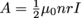, where r is the radius from the center of the solenoid. This equation only works within the solenoid. In the absolute center of the solenoid, the potential is 0, as seen below.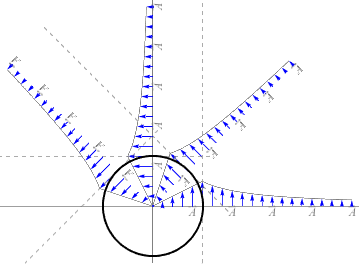Just as with electric fields, the lines of magnetic equipotential are perpendicular to the magnetic field lines, another symmetry with electricity. Unlike electric potential, the magnetic potential is a vector quantity with a direction and not just a number.

Not much meat offered about magnetic potential, but who wants more calculus? Not us.

### Common Mistakes

Double check the constants and their meanings in an equation: many symbols have multiple meanings in electricity and magnetism, which makes it harder to keep track yet keeps us on our toes.

Also, don’t confuse the vector potential A with the scalar potential V. The scalar potential is electric potential, which is the work needed to move a positive electric charge q from infinity to a point in an electric field E.

### Brain Snack

Earth has a magnetic dipole moment of 7.94 × 1022 A m2. The electron, on the other hand, has a magnetic dipole moment of 9.28 × 10-24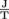, which are equivalent units to A m2. That’s an order of 46 orders of magnitude difference between a solitary electron and our grand planet.